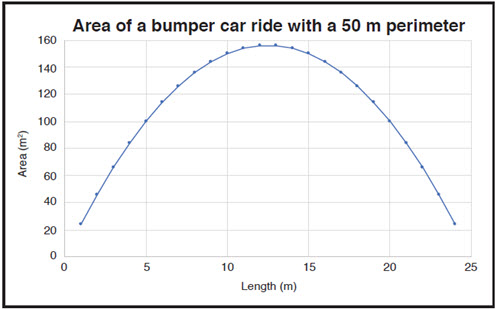Go to website

# reSolve: Area and Perimeter

This series of three lessons explores the relationship between area and perimeter using the context of bumper cars at an amusement park. Students design a rectangular floor plan with the largest possible area with a given perimeter. They then explore the perimeter of a bumper car ride that has a set floor area and investigate the possible rectangles that result. They graph their results and explore the concept that the smaller the difference in side lengths, the smaller the perimeter. Students then design three different floor plans for bumper car rides with different criteria. The lesson is outlined in detail including curriculum links, vocabulary, materials needed, presentation slides, discussion points and student resources. This sequence is part of the reSolve: Mathematics by Inquiry program.

Year level(s) Year 5
Audience Teacher
Purpose Teaching resource
Teaching strategies and pedagogical approaches Mathematics investigation
Keywords factors, perimeter, rectangles, length, area

## Curriculum alignment

Curriculum connections Numeracy
Strand and focus Number, Measurement, Build understanding, Apply understanding
Topics Area, volume and surface area, Multiples, factors and powers
AC: Mathematics (V9.0) content descriptions
AC9M5N02
Express natural numbers as products of their factors, recognise multiples and determine if one number is divisible by another

AC9M5N07
Solve problems involving division, choosing efficient strategies and using digital tools where appropriate; interpret any remainder according to the context and express results as a whole number, decimal or fraction

Numeracy progression Multiplicative strategies (P6, P7)
Interpreting fractions (P8)